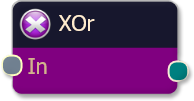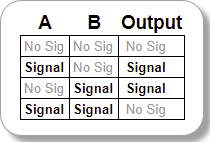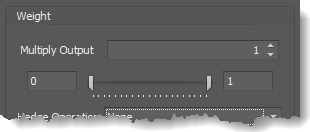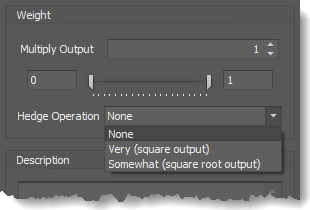#### Risk Disclosure

Futures, foreign currency and options trading contains substantial risk and is not for every investor. An investor could potentially lose all or more than the initial investment. Risk capital is money that can be lost without jeopardizing ones financial security or lifestyle. Only risk capital should be used for trading and only those with sufficient risk capital should consider trading. Past performance is not necessarily indicative of future results. View full risk disclosure.

## XOr NodeThe XOr logic node applies a Boolean XOr operation.  When an XOr has two inputs, it performs a logical operation that outputs a signal only when both inputs differ (one input has a signal, the other has No signal).  In other words the output is a signal if the inputs are not alike, otherwise the output is false.  A way to remember XOR is “one or the other, but not both”.When an XOr has three or more inputs, the logical result outputs a signal when the number of signals at the inputs is odd, and no output signal when the number of input signals is even.

Reminder:  Long and Short are calculated separately in BloodHound.  i.e. In the example of an XOr, if you have two solvers connected and one is producing a Long Signal and the other is producing a Short Signal, this will result in a signal in both directions.  See the BloodHound Output section for more information on the Long and Short separate calculations.

# BloodHound   v2

This section is specific to BloodHound v2 Property Inspector's elements.  The node functions exactly the same in BloodHound v1 and v2.

## Properties tab

This tab contains the essential settings for all nodes.

### Modifiers

Please note:   The buttons only modify the individual instance of the selected node(s) on a Logic Board.   Thus, the buttons are only visible when a node is selected on the Logic Board.  The buttons are not available when a solver is selected in the Solvers panel, because the original solver's output can not be globally modified.
This behavior is consistent with adding a function node afterwards, so that the original solver's output remains unmodified elsewhere in the system.  It is similar to having an SMA(50) on several charts.  Changing the plot color on one chart does not modify the plot color on the other charts.

## Options tab

This tab contains extra secondary settings common to all nodes.

### Weight

This section is used to modify the node's final output value.  It is only useful for designing fuzzy logic systems, or a fuzzy logic section of a system.
Please note:   The Weight controls only modify the individual instance of the selected node(s) on a Logic Board.   Thus, the Weight controls are only visible when a node is selected on the Logic Board.  The controls are not available when a solver is selected in the Solvers panel, because the original solver's output can not be globally modified.
This behavior is different than BloodHound 1.0.  In BH 1.0, the Weight controls modified the nodes output globally (every instance).  This change in BH 2.0 adds more system design granularity.Multiply Output:   This takes the internal values (the Long and Short values determined via the Properties tab » Output Rules section) and multiplies them by this value.  Note: the final output will not exceed a value of 1, as described in the Slider control below.

Slider control:  The slider constrains the final output to a value of 0 to 1.  The left side of the slider sets a minimum value that is output regardless if the solver condition is true or not.  The right side sets a maximum value that is output.  The output is capped.
e.g.  Three indicator conditions are being checked, and thus three solvers are created.  Only two out of the three indicator conditions are needed.  An Additive logic node is used to add the solver's outputs together.  Just two out of the three solvers need to add up to a value of 1.  Therefore, the right slider (max output value) for all three solvers is set to 0.5.  When two indicator/solver conditions are found, thus the outputs = 0.5, then the calculation, in the Additive node, is 0.5 + 0.5 + 0 = 1.  A value of 1 means the two out of three condition is true.Hedge Operation: This applies a mathematical formula to the internal value.

None:  No modification is applied.
Very (square output):  A squaring formula is applied.  Output  =  value .
Somewhat (square root output):  A square root formula is applied.  Output  =  √value .

### Description

This text area provides a place to write a full description of what the node is doing, used for, or what ever you want.
Note:   The Description is global to all instances of the node.  It is not applied individually to each instance as the Weight controls are.

## Help tab

This tab displays the documentation page (from this web site) of the selected node.
Please note:  NinjaTrader v8.0.26.0 or newer is required for the built in web viewer to work, and thus the documentation to be displayed.

#### Risk Disclosure

Futures, foreign currency and options trading contains substantial risk and is not for every investor. An investor could potentially lose all or more than the initial investment. Risk capital is money that can be lost without jeopardizing ones financial security or lifestyle. Only risk capital should be used for trading and only those with sufficient risk capital should consider trading. Past performance is not necessarily indicative of future results. View full risk disclosure.

# XOr NodeThe XOr logic node applies a Boolean XOr operation.  When an XOr has two inputs, it performs a logical operation that outputs a signal only when both inputs differ (one input has a signal, the other has No signal).  In other words the output is a signal if the inputs are not alike, otherwise the output is false.  A way to remember XOR is “one or the other, but not both”.When an XOr has three or more inputs, the logical result outputs a signal when the number of signals at the inputs is odd, and no output signal when the number of input signals is even.

Reminder:  Long and Short are calculated separately in BloodHound.  i.e. In the example of an XOr, if you have two solvers connected and one is producing a Long Signal and the other is producing a Short Signal, this will result in a signal in both directions.  See the BloodHound Output section for more information on the Long and Short separate calculations.

The XOr node in BloodHound v1 and v2 function exactly the same.  The only difference is how the node is visualized in the Property Inspector.  See the BloodHound v2 section above for a description and example.

#### Risk Disclosure

Futures, foreign currency and options trading contains substantial risk and is not for every investor. An investor could potentially lose all or more than the initial investment. Risk capital is money that can be lost without jeopardizing ones financial security or lifestyle. Only risk capital should be used for trading and only those with sufficient risk capital should consider trading. Past performance is not necessarily indicative of future results. View full risk disclosure.

## XOr NodeThe XOr logic node applies a Boolean XOr operation.  When an XOr has two inputs, it performs a logical operation that outputs a signal only when both inputs differ (one input has a signal, the other has No signal).  In other words the output is a signal if the inputs are not alike, otherwise the output is false.  A way to remember XOR is “one or the other, but not both”.When an XOr has three or more inputs, the logical result outputs a signal when the number of signals at the inputs is odd, and no output signal when the number of input signals is even.

Reminder:  Long and Short are calculated separately in BloodHound.  i.e. In the example of an XOr, if you have two solvers connected and one is producing a Long Signal and the other is producing a Short Signal, this will result in a signal in both directions.  See the BloodHound Output section for more information on the Long and Short separate calculations.